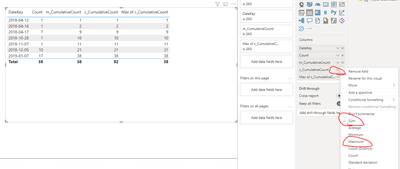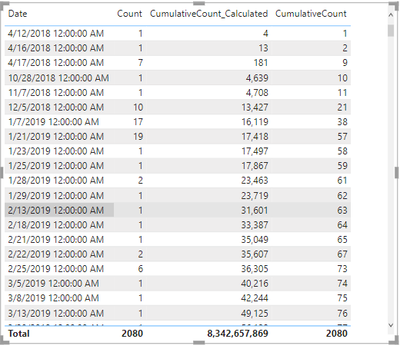cancel
Showing results for
Did you mean:Frequent Visitor

## Create a calculated column for cumulative sum by Date

I'm trying to create a calculated column that calculates the cumulative sum. I created one in a DAX measure and its working fine:

CumulativeCount =
CALCULATE(
SUM(Fact_CRM_CT[Count]),
FILTER(ALLSELECTED(Fact_CRM_CT),Fact_CRM_CT[DateKey] <= MAX(Fact_CRM_CT[DateKey]) ))

I want to recreate the same in a calculated column but it is not working as expected.
1 ACCEPTED SOLUTIONCommunity Support

Hi, @Aymane

You are using a calculated column instead of measure column to display the result, so the final result is aggregated without recomputing.

You just need to change the summarize type of  calculated column 'Cumulative Count' from 'Sum' to 'Maximum'/'Minimum'/'Average'.Best Regards,
Community Support Team _ Eason

3 REPLIES 3Frequent Visitor

Hi @amitchandak,

I have tried your solution but it still doesn't give the correct output as you can see below:Community Support

Hi, @Aymane

You are using a calculated column instead of measure column to display the result, so the final result is aggregated without recomputing.

You just need to change the summarize type of  calculated column 'Cumulative Count' from 'Sum' to 'Maximum'/'Minimum'/'Average'.Best Regards,
Community Support Team _ EasonSuper User

@Aymane , a new column

CumulativeCount =
CALCULATE(
SUM(Fact_CRM_CT[Count]),
FILTER((Fact_CRM_CT),Fact_CRM_CT[DateKey] <= earlier(Fact_CRM_CT[DateKey]) ))

!! Microsoft Fabric !!
Microsoft Power BI Learning Resources, 2023 !!
Learn Power BI - Full Course with Dec-2022, with Window, Index, Offset, 100+ Topics !!
Did I answer your question? Mark my post as a solution! Appreciate your Kudos !! Proud to be a Super User! !!